# 2021数学建模国赛B题复盘详细解析

## 题目

C4 烯烃广泛应用于化工产品及医药的生产，乙醇是生产制备 C4 烯烃的原料。在制备过程中，催化剂组合（即：Co 负载量、Co/SiO2 和 HAP 装料比、乙醇浓度的组合）与温度对 C4 烯烃的选择性和 C4 烯烃收率将产生影响（名词解释见附录）。因此通过对催化剂组合设计，探索乙醇催化偶合制备 C4 烯烃的工艺条件具有非常重要的意义和价值。

### 名词解释与附件说明## 第一问

### 分析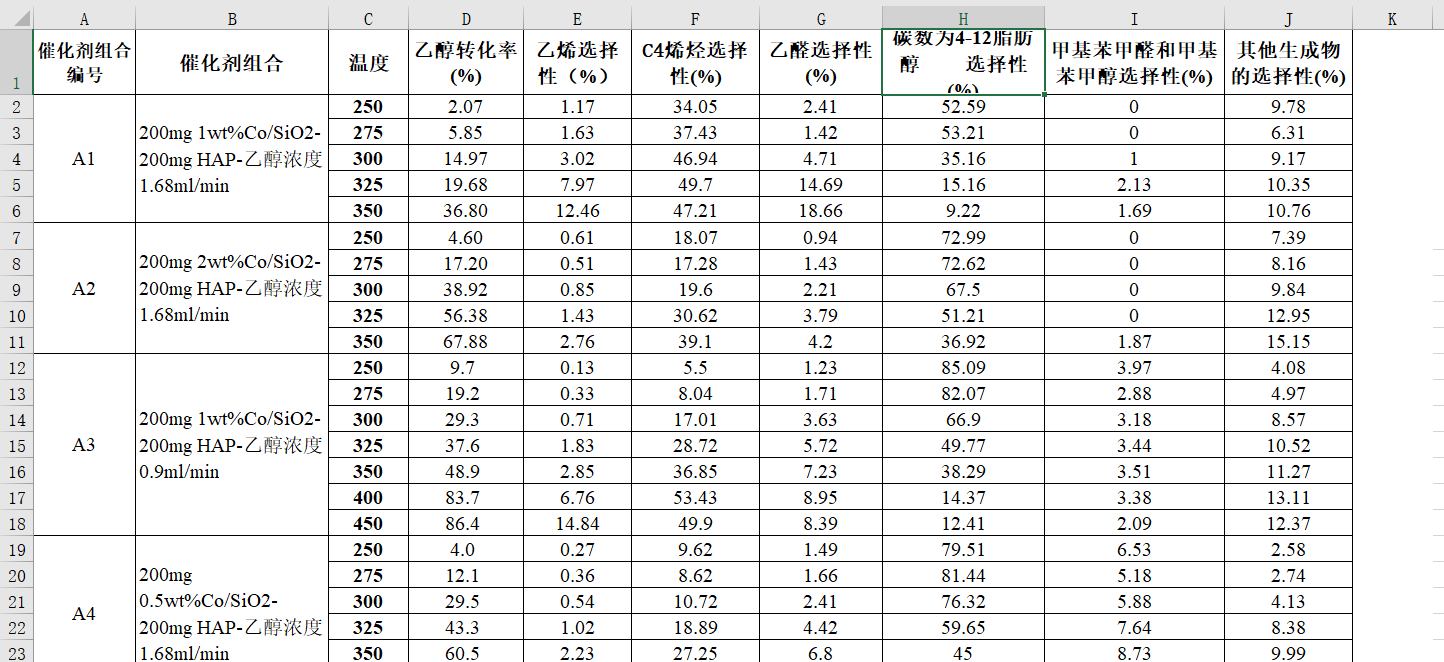``````200mg 1wt%Co/SiO2- 200mg HAP-乙醇浓度1.68ml/min
``````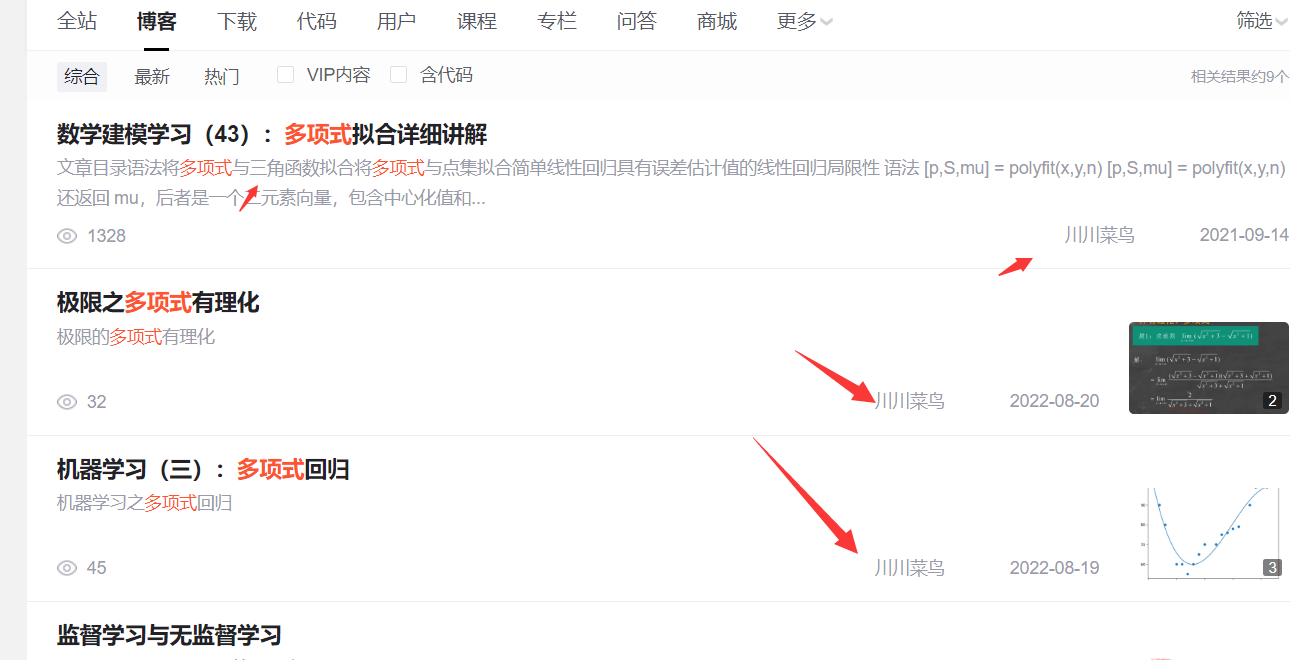### 第一小问

``````import numpy as np
import matplotlib.pyplot as plt

x=np.array([250,275,300,325,350]).reshape((-1, 1))
y=np.array([2.07,5.85,14.97,19.68,36.80])

plt.scatter(x, y) # 绘制散点图
plt.show()
``````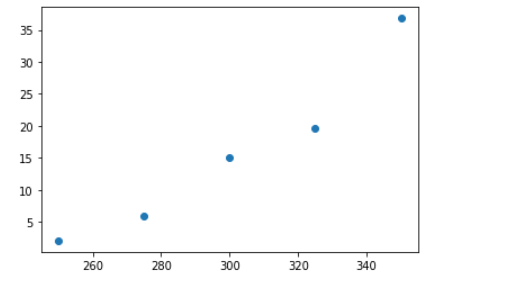``````from sklearn.linear_model import LinearRegression
#实例化线性模型
lr = LinearRegression()
lr.fit(x, y)
y_predict = lr.predict(x)

plt.scatter(x, y )
plt.plot(x, y_predict)
````````````import numpy
x=[250,275,300,325,350]
y=[2.07,5.85,14.97,19.68,36.80]

mymodel = numpy.poly1d(numpy.polyfit(x, y, 4)) # 三阶

myline = numpy.linspace(250, 350, 100) #

plt.scatter(x, y) # 原始点
plt.plot(myline, mymodel(myline)) # 多项式回归
plt.show() #显示
``````A3拟合：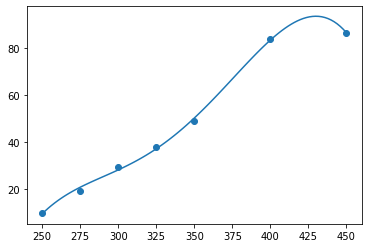A4拟合：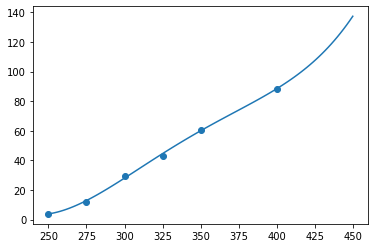``````print(numpy.polyfit(x4, y4, 4))
``````

[ 2.36276730e-07 -3.22254088e-04 1.63402013e-01 -3.58919245e+01
2.87645755e+03]

``````import pandas as pd

x4=[250,275,300,325,350,400]
y4=[4.0,12.1,29.5,43.3,60.5,88.4]

data = [[250,4],[275,12.1],[300,29.5],[325,43.3],[350,60.5],[400,88.4]]

df = pd.DataFrame(data,columns=['x1','x2']) # 将第一维度数据转为为行，第二维度数据转化为列，即 3 行 2 列，并设置列标签

c1=df['x1']
c2=df['x2']

result= np.corrcoef(c1,c2)
result
``````

``````array([[1.        , 0.99751423],
[0.99751423, 1.        ]])
``````

``````import matplotlib.pyplot as plt
figure, ax = plt.subplots(figsize=(12, 12))
sns.heatmap(df.corr(), square=True, annot=True, ax=ax)
``````### 第二小问

``````x=[250,275,300,325,350]
z=[34.05,37.43,46.94,49.7,47.21]

model = numpy.poly1d(numpy.polyfit(x, z, 4)) # 4阶

line = numpy.linspace(250, 350, 1000)

plt.scatter(x, z) # 原始点
plt.plot(line, model (line)) # 多项式回归
plt.show() #显示
``````### 第三小问``````import matplotlib.pyplot as plt
plt.rcParams['font.sans-serif'] = ['SimHei'] # 中文

fig = plt.figure(figsize=(7, 4),dpi=150)  # 大小和清晰度

ax1.plot(x, y1)
ax1.set_ylabel('乙醇转化率')
ax1.set_title("350℃下乙醇转化率和各种生成物的选择性随时间变化曲线")

ax2 = ax1.twinx()

ax2.plot(x, y2)
ax2.plot(x, y3)
ax2.plot(x, y4)
ax2.plot(x, y5)
ax2.plot(x, y6)

ax2.set_ylabel('选择性')

plt.legend(data.columns,fontsize = 10) # 图标
plt.savefig(r"双y轴.png")
plt.show()
``````## 第二问

### 拓展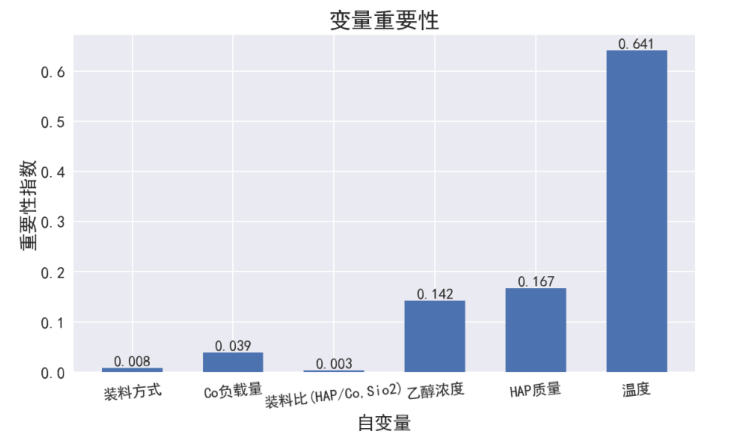## 第三问

### 分析``````y =10238-33.5x1一64.7T＋0.1T^2+0.1x1*T-0.7x4*T
``````### 拓展``````𝑦1 = −70.3901 − 16.2233𝑋1 − 4.0639𝑋2 + 0.0979𝑋3 + 0.3339𝑋4 − 8.4602𝑋5
− 6.2275𝑋6
``````

C4 烯烃选择性为：

``````𝑦2 = −30.845 − 349𝑋1 − 11.8455𝑋2 + 0.0753𝑋3 + 0.1819𝑋4 + 3.1166𝑋5
− 3.4989𝑋6
``````

``````𝑦 = 𝑦1 · 𝑦2
``````